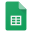# Score Function optimization

Formula assignments requires use of a score which need to be computed using a set of positive and negative weights. To optimize these weights, we can use a set of highest quality annotations using authentic standards.

Provide the parameters in this excel file and then below code.

library(IDSL.UFA)

UFA_workflow(spreadsheet = "name of the parameter excel file")

# it will suggest a set of five coefficients that may be helpful in improving the formula assignments for different instruments and samples.UFA_parameters_score_functiona_optimization.xlsx